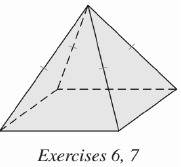Chapter 9.CT, Problem 6CTElementary Geometry For College St...

7th Edition
Alexander + 2 others
ISBN: 9781337614085

Solutions

Chapter
SectionElementary Geometry For College St...

7th Edition
Alexander + 2 others
ISBN: 9781337614085
Textbook Problem

Find the height of the regular square pyramid shown if each edge of the base measures 8 in. and the length of the slant height is 5 in.To determine

To find:

The height of the regular square pyramid.

Explanation

Given:

For a regular square pyramid with each edge of the base measures 8 in. and the length of the slant height is 5 in.

Formula used:

In a regular pyramid, l2=a2+h2 where a is the apothem and h is the height.

Calculation:

We know that the length of the apothem of the square is one half of the length of the edge.

Therefore a=82=4 in

We have l=5 in

Thus l2=a2+h2 becomes

Still sussing out bartleby?

Check out a sample textbook solution.

See a sample solution

The Solution to Your Study Problems

Bartleby provides explanations to thousands of textbook problems written by our experts, many with advanced degrees!

Get Started

Find more solutions based on key concepts